# 传染病模型

• ## 基础定义

1. $S$(Susceptible)类：指未得病，但与感染者接触后容易收到感染的人。
2. $E$(Exposed)类：指接触过感染者，但暂时没有传播的能力的人。
3. $I$(Infectious)类：指染上传染病，具有传播能力的人。（可以传播给$S$类人员，将其变成$E$类或$I$类成员）
4. $R$(Recovered / Removed)类：指病愈而具有免疫力的人或被隔离的移出者。（如果免疫期有限，$R$类人员可以重新变为$S$类）
• ## 常见模型

### 1.1 模型假设

1. 将人群分为$S$类和$I$类，在疾病传播期内所考察地区的总人数$K$不变（即不考虑生死和迁移）。时刻$t$这两类人群人数分别记为$S(t)$$I(t)$
2. 每个传染病患者每天有效接触的平均人数为$\beta$（称为日接触率）。当传染病患者与健康人接触会将健康人感染为传染病患者。
3. 初始时刻传染病患者人数为$I_0$

### 1.2 模型建立

$\begin{array}{c} {dI(t) \over dt} = \beta I(t){S(t) \over K} \\ S(t) + I(t) = K \\ I(0) = I_0 \\ \end{array}$

### 1.3 模型求解

$\begin{array}{c} {dI(t) \over dt} = \beta I(t)(1 - {I(t) \over K}) \end{array}$

$\begin{array}{c} {d{I(t) \over K} \over dt} = \beta {I(t) \over K}(1 - {I(t) \over K}) \end{array}$

${i(t) = {I(t) \over K} }$，则${i(t)}$称为感染率。得：

$\begin{array}{c} {di(t) \over dt} = \beta i(t)(1 - i(t)) \\ i_0 = i(0) = {I_0 \over K} \end{array}$

$\begin{array}{c} i(t) = {1 \over {1 + e^{- \beta t}({1 \over i_0} - 1)} } \end{array}$

${i(t)}$ ~ $t$${di(t) \over dt}$ ~ ${i(t)}$的图像如下：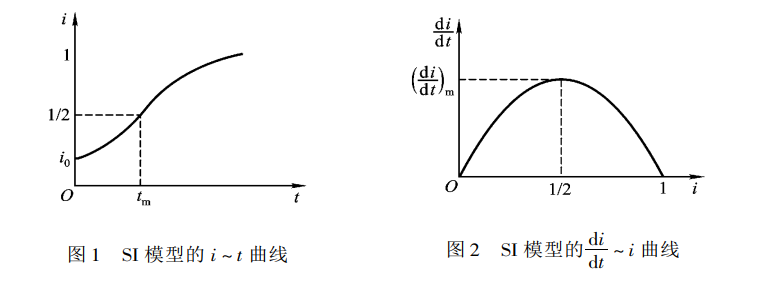$\begin{array}{c} t_m = {\beta}^{-1}ln({1 \over i_0} - 1) \end{array}$

### 1.4 模型假设

1. 将人群分为$S$类和$I$类，在疾病传播期内所考察地区的总人数$K$不变（即不考虑生死和迁移）。时刻$t$这两类人群人数分别记为$S(t)$$I(t)$
2. 每个传染病患者每天有效接触的平均人数为$\beta$（称为日接触率）。当传染病患者与健康人接触会将健康人感染为传染病患者。
3. 每天被治愈的患者数占总患者的比率为常熟$\mu$（称为日治愈率）。病人治愈后成为仍可被感染的健康人。易知${1 \over \mu}$为传染病的平均传染期。
4. 初始时刻传染病患者人数为$I_0$

### 1.5 模型建立

$\begin{array}{c} {dI(t) \over dt} = \beta I(t){S(t) \over K} - \mu I(t) \\ S(t) + I(t) = K \\ I(0) = I_0 \end{array}$

### 1.6 模型求解

$\begin{array}{c} {dI(t) \over dt} = \beta I(t)(1 - {I(t) \over K}) - \mu I(t) \end{array}$

$\begin{array}{c} {d{I(t) \over K} \over dt} = \beta {I(t) \over K}(1 - {I(t) \over K}) - \mu {I(t) \over K} \end{array}$

${i(t) = {I(t) \over K} }$，则${i(t)}$称为感染率。得：

$\begin{array}{c} {di(t) \over dt} = \beta i(t)(1 - i(t)) - \mu i(t) \end{array}$

${\sigma = {\beta \over \mu} }$，可知$\sigma$是整个传染期内每个患者有效接触的平均人数，称为接触数。得：

$\begin{array}{c} {di(t) \over dt} = -\beta i(t)(i(t) - (1 - {1 \over \sigma})) \\ i_0 = i(0) = {I_0 \over K} \end{array}$

### 3.1 模型假设

1. 将人群分为健康人（$S$类）、传染病患者（$I$类）和病愈/死亡的移出者（$R$类）（免疫期近似$\infty$），在疾病传播期内所考察地区的总人数$K$不变。时刻$t$这三类人群人数分别记为$S(t)$$I(t)$$R(t)$
2. 传染病患者的日接触率为$\beta$，日治愈率为$\mu$，传染期接触的总人数为${\sigma = {\beta \over \mu} }$
3. 初始时刻的健康人数和患者数分别为${S_0}$${I_0}$

### 3.2 模型建立

$\begin{array}{c} {dS(t) \over dt} = -\beta I(t){S(t) \over K} \\ {dI(t) \over dt} = \beta I(t){S(t) \over K} - \mu I(t) \\ S(t) + I(t) + R(t) = K \\ S(0) = S_0 \\ I(0) = I_0 \end{array}$

${s(t) = {S(t) \over K} }$，则${s(t)}$称为未感染率；令${i(t) = {I(t) \over K} }$，则${i(t)}$称为感染患病率；令${r(t) = {R(t) \over K} }$，则${r(t)}$称为移出率。

$\begin{array}{c} {ds(t) \over dt} = -\beta i(t)s(t) \\ {di(t) \over dt} = \beta i(t)s(t) - \mu i(t) \\ s(t) + i(t) + r(t) = 1 \\ s_0 = s(0) = {S_0 \over K} \\ i_0 = i(0) = {I_0 \over K} \end{array}$

### 3.3 模型仿真

${K = 10000}$${\beta = 1}$${\mu = 0.3}$${s_0 = 0.98}$${i_0 = 0.02}$

1. 利用AnyLogic仿真软件仿真模型如下：
• 仿真数据：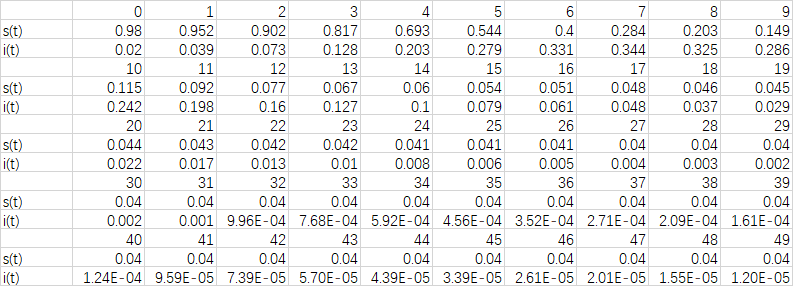• 仿真图像：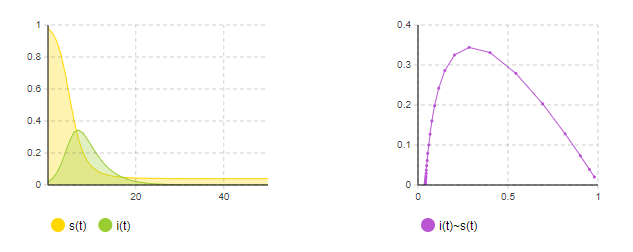1. 利用matlab仿真模型如下：
• matlab代码：
• 仿真数据：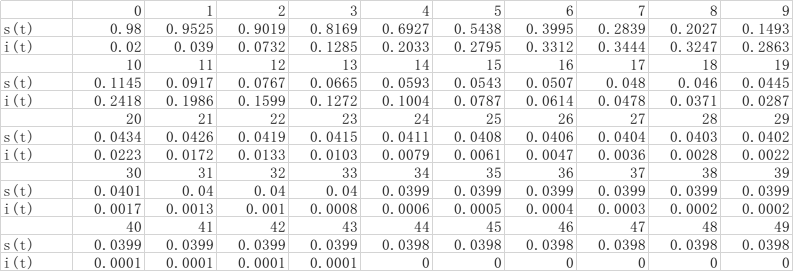• 仿真图像：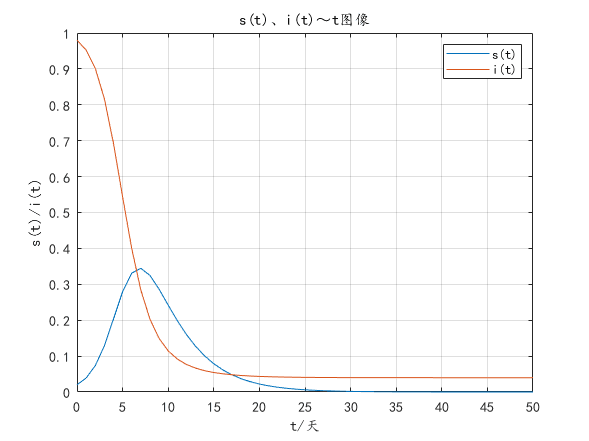s(t)、i(t)与t的图像 i(t)与s(t)的图像

### 3.4 模型分析

1. 相轨线定义（摘自百度百科——相轨线
对于微分方程：

$\begin{array}{c} {dx \over dt} = P(t, x, y) \\ {dy \over dt} = Q(t, x, y) \\ x(t_0) = x_0 \\ y(t_0) = y_0 \end{array}$

$\begin{array}{c} x = x(t) \\ y = y(t) \end{array}$

1. 相轨线分析
由以上可知，${s}$~${i}$平面成为相平面，相轨线在相平面上的定义域为：

$\begin{array}{c} D = \{ (s, t) | \, s \geq 0, i \geq 0, {s + i} \leq 1 \} \end{array}$

${\sigma = {\beta \over \mu} }$（含义同SIS模型）。

$\begin{array}{c} {ds(t) \over dt} = -\beta i(t)s(t) \\ {di(t) \over dt} = \beta i(t)s(t) - \mu i(t) \end{array}$

$\begin{array}{c} {di \over ds} = {1 \over \sigma s} - 1 \\ i|_{s=s_0} = i_0 \end{array}$

$\begin{array}{c} i = (s_0 + i_0) - s + {1 \over \sigma} ln{s \over s_0} \end{array}$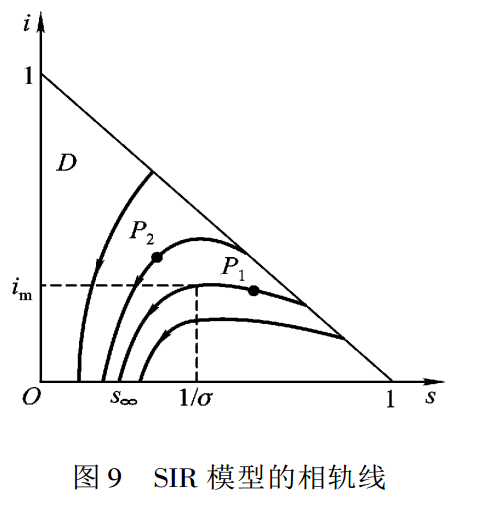• 不论初始条件${s_0}$${i_0}$如何，病人终将消失，即

$\begin{array}{c} i_\infty = 0 \end{array}$

• 最终未被感染的健康者的比例是${s_\infty}$${s_\infty}$是方程

$\begin{array}{c} s_0 + i_0 - s_\infty + {1 \over \sigma}ln{s_\infty \over s} = 0 \end{array}$

${(0, {1 \over \sigma})}$内的根。

• ${s_0 \gt {1 \over \sigma} }$，则${i(t)}$先增加后减小；当${s = {1 \over \sigma} }$时，${i(t)}$达到最大值

$\begin{array}{c} i_m = s_0 + i_0 - {1 \over \sigma} \end{array}$

• ${s_0 \leq {1 \over \sigma} }$，则${i(t)}$单调减小至零，${s(t)}$单调减小至${s_\infty}$

${\sigma = {\beta \over \mu} }$中，人们的卫生水平越高，日接触率$\beta$越小；医疗水平越高，日治愈率$\mu$越大。可知，提高医疗卫生水平有助于控制传染病的蔓延。

### 4.1 模型假设

1. 将人群分为健康人（$S$类）、传染病患者（$I$类）和病愈的移出者（$R$类）（免疫期近似$\infty$），在疾病传播期内所考察地区的总人数$K$不变。时刻$t$这三类人群人数分别记为$S(t)$$I(t)$$R(t)$
2. 传染病患者的日接触率为$\beta$，日治愈率为$\mu$，传染期接触的总人数为${\sigma = {\beta \over \mu} }$
3. 初始时刻的健康人数和患者数分别为${S_0}$${I_0}$